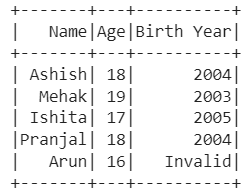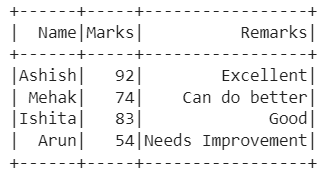GeeksforGeeks App
Open AppBrowser
Continue

# PySpark UDFs with List Arguments

Are you a data enthusiast who works keenly on Python Pyspark data frame? Then, you might know how to link a list of data to a data frame, but do you know how to pass list as a parameter to UDF? Don’t know! Read the article further to know about it in detail.

## PySpark – Pass list as parameter to UDF

First of all, import the required libraries, i.e., SparkSession, SQLContext, UDF, col, StringType. The SparkSession library is used to create the session, while the SQLContext is used as an entry point to SQL in Python. The UDF is used to create a reusable function in Pyspark, while col is used to return a column based on the given column name. Also, the StringType is used to represent character string values. Now, create a spark session using getOrCreate function. Then, create spark and SQL contexts too. Further, create a data frame using createDataFrame function. Moreover, create a list that has to be passed as a parameter. Now, create a function which will be called to pass a list as a default value to a variable. In this function, assign the values from the list using the if else condition. Later on, create a user-defined function with parameters as a function created and column type. Finally, create a new column by calling the user-defined function, i.e., UDF created and displays the data frame,

### Example 1:

In this example, we have created a data frame with two columns ‘Name‘ and ‘Age‘ and a list ‘Birth_Year‘. Further, we have created a function which will pass a list as a variable and then call that function as an argument in UDF to display the updated data frame.

## Python3

 `# Import the libraries SparkSession, SQLContext, UDF, col, StringType``from` `pyspark.sql ``import` `SQLContext, SparkSession``from` `pyspark.sql.functions ``import` `udf, col``from` `pyspark.sql.types ``import` `StringType`` ` `# Create a spark session using getOrCreate() function``spark_session ``=` `SparkSession.builder.getOrCreate()`` ` `# Create a spark context``sc ``=` `spark_session.sparkContext`` ` `# Create a SQL context``sqlContext ``=` `SQLContext(sc)`` ` `# Create the data frame using createDataFrame function``data_frame ``=` `sqlContext.createDataFrame(``    ``[(``"Ashish"``, ``18``), (``"Mehak"``, ``19``), (``"Ishita"``, ``17``),``     ``(``"Pranjal"``, ``18``), (``"Arun"``, ``16``)], [``"Name"``, ``"Age"``])`` ` `# Create a list to be passed as parameter``Birth_Year ``=` `[``"2004"``, ``"2003"``, ``"2005"``]`` ` `# Create a function to pass list as default value to a variable`` ` ` ` `def` `parameter_udf(age_index, label``=``Birth_Year):``    ``if` `age_index ``=``=` `18``:``        ``return` `label[``0``]``    ``elif` `age_index ``=``=` `17``:``        ``return` `label[``2``]``    ``elif` `age_index ``=``=` `19``:``        ``return` `label[``1``]``    ``else``:``        ``return` `'Invalid'`` ` ` ` `# Create a user defined function with parameters``# parameter_udf and column type.``udfcate ``=` `udf(parameter_udf, StringType())`` ` `# Create a column by calling the user defined function``# created above and display data frame``data_frame.withColumn(``"Birth Year"``, udfcate(``"Age"``)).show()`

Output:### Example 2:

In this example, we have created a data frame with two columns ‘Name‘ and ‘Marks‘ and a list ‘Remarks_List‘. Further, we have created a function which will pass a list as a variable and then call that function as an argument in UDF to display the updated data frame.

## Python3

 `# Import the libraries SparkSession, SQLContext, UDF, col, StringType``from` `pyspark.sql ``import` `SQLContext, SparkSession``from` `pyspark.sql.functions ``import` `udf, col``from` `pyspark.sql.types ``import` `StringType`` ` `# Create a spark session using getOrCreate() function``spark_session ``=` `SparkSession.builder.getOrCreate()`` ` `# Create a spark context``sc``=``spark_session.sparkContext`` ` `# Create a SQL context``sqlContext``=` `SQLContext(sc)`` ` `# Create the data frame using createDataFrame function``data_frame``=` `sqlContext.createDataFrame([(``"Ashish"``, ``92``),``                                        ``(``"Mehak"``, ``74``),``                                        ``(``"Ishita"``, ``83``),``                                        ``(``"Arun"``,``54``)],``                                       ``[``"Name"``, ``"Marks"``])`` ` `# Create a list to be passed as parameter``Remarks_List ``=` `[``"Excellent"``, ``"Good"``, ``"Can do better"``]`` ` `# Create a function to pass list as default value to a variable``def` `parameter_udf( marks_index,label``=``Remarks_List):``    ``if` `marks_index >``85``:``        ``return` `label[``0``]``    ``elif` `marks_index > ``75``:``        ``return` `label[``1``]``    ``elif` `marks_index >``60``:``        ``return` `label[``2``]``    ``else``: ``        ``return` `'Needs Improvement'`` ` `# Create a user defined function with parameters ``# parameter_udf and column type.``udfcate ``=` `udf(parameter_udf, StringType())`` ` `# Create a column by calling the user defined function ``# created above and display data frame``data_frame.withColumn(``"Remarks"``, udfcate(``"Marks"``)).show()`

Output:My Personal Notes arrow_drop_up